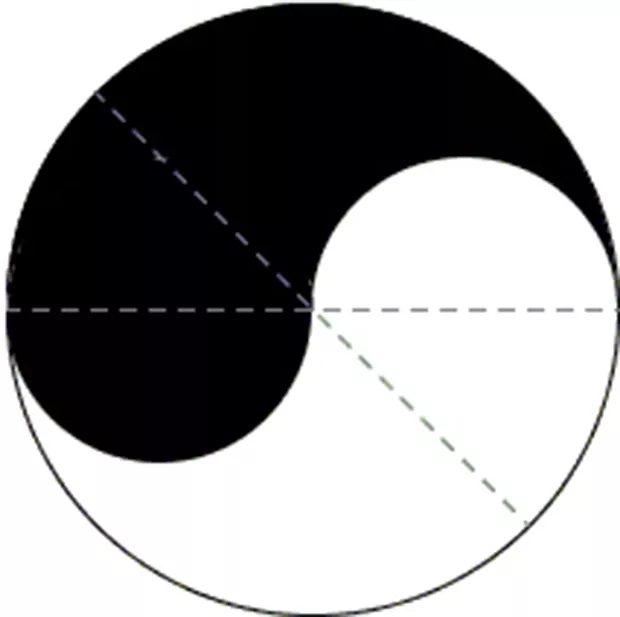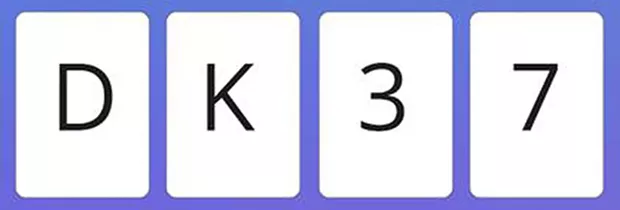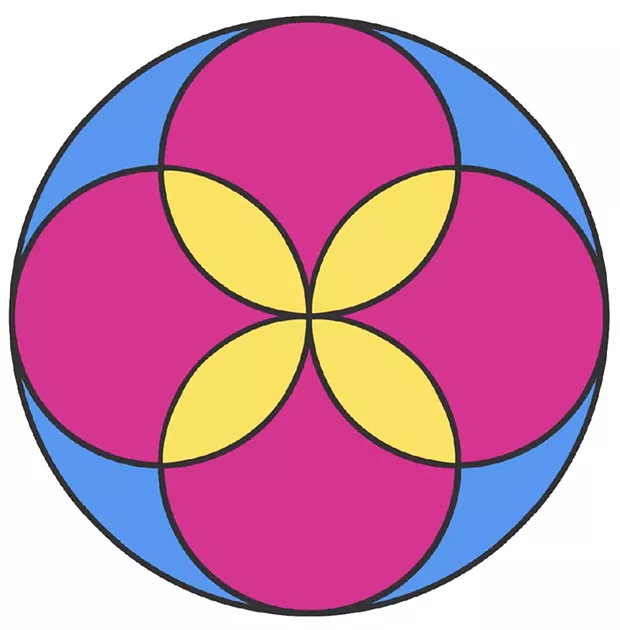# Seven Puzzles for the Seventh Month

Page 2 of 2

The Old Bridge

Alice and Bill cross (two minutes elapsed); Alice returns with the flashlight (three minutes); Cassie and Dave cross (13 minutes); Bill returns with the flashlight (15 minutes); Alice and Dave cross (17 minutes total).

Gender Gap

(c), since every birth has a 50/50 chance of being male. (Past results have no effect on the probability of the next child's gender.)

Yin and Yang

The small white and black semicircles above and below the horizontal line each have 1/8 of the area of the whole (since the area of a circle is proportional to its diameter squared), as do the two triangles (45 degrees being 1/8 of a full circle). Hence the white area above the 45 degree line has ¼ the area of the whole circle. By symmetry, so does the black area below the 45 degree line. Therefore the 45 degree line bisects both the white and black shapes.Four Cards

Cards 1 and 4. Obviously, you have to check card 1 to see if there's a 3 on the back. Card 2 is irrelevant. So is card 3, since whether there's a D or not doesn't affect the validity of the statement. But if there's a D on the back of card 4, the statement is false. (In a test of this logic puzzle, devised by psychologist Peter Wason in 1966, only 10 percent of the general public and 43 percent of mathematicians got it right!)Harry S Truman

S

Percentages

(e) (1 divided by 1/10 = 10)

Circles

(b) Each small circle has 1/4 the area of the big circle, so their total area is equal to that of the big circle. Thus, the total area of the overlaps (yellow) must be equal to the total area not covered by the small circles (blue).Bonus

All are pseudonyms: Ellis Bell was Emily Bronte, Ann Landers was Ruth Crowley (later, "Eppie" Lederer), Robert Galbraith is J.K. Rowling, Anne Rice is Howard Allen O'Brien, George Orwell was Eric Arthur Blair.